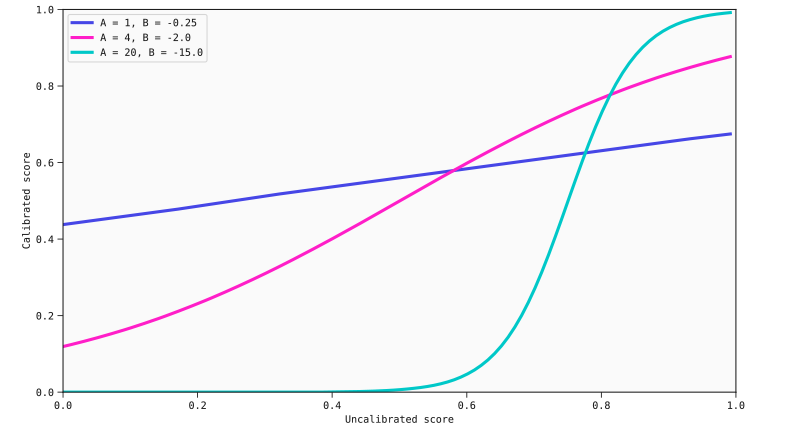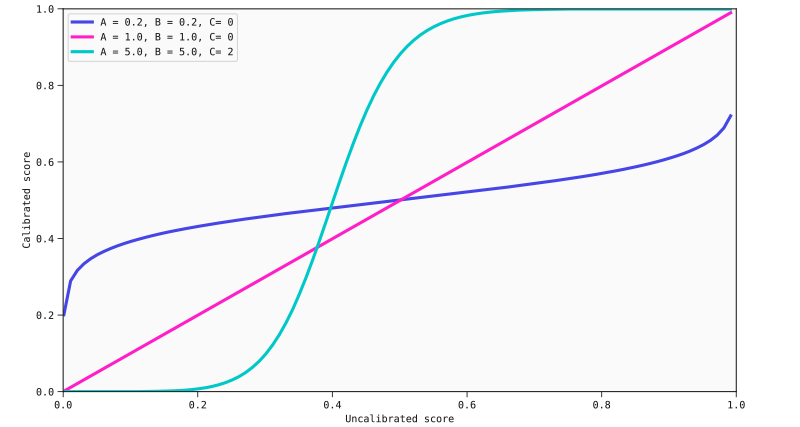# An introduction to calibration (part II): Platt scaling, isotonic regression, and beta calibration

Calibrators are tools used to transform the scores generated by your models into (almost) real mathematical probabilities.

In the first part of this blog series we learnt the basics of how to evaluate the calibration of a model, but we didn’t address what you can do to improve it in this regard.

The goal of this blog post is to introduce you to calibrators, which are tools that you can use to transform the scores generated by your models into something that is as close as possible to real mathematical probabilities.

## Platt scaling

One of the most popular calibrators is Platt scaling (or logistic regression). It was originally proposed by John C. Platt in 2000 , and it consists in fitting the scores s_i given by the uncalibrated model for the positive class to the sigmoid

where the parameters A > 0 and B are obtained by minimising the log-loss for a given training set.Some of the calibration maps that can be obtained through Platt scaling.

Being a parametric model (which makes assumptions about the score distributions), Platt scaling has the advantage that it does not require a lot of data to be trained. But in some cases this has significant drawbacks.

As discussed in Beyond sigmoids , this method can lead to quite good calibrations as long as the ratio of the score distributions for both binary classes is similar to the ratio of two Gaussian distributions with equal variance. Unfortunately, if this is not the case, Platt scaling can actually lead to even worse calibrated scores, as the identity function is not present in the family of functions that can be obtained from (1), which means it would leave the scores untouched if there is no better calibration.

## Isotonic regression

Isotonic regression (Zadrozny, Elkan, 2002 ) is a calibration method that provides a discrete, step-wise, monotonically increasing calibration map consisting of a set of points arranged in a staircase-like way. The calibration map for isotonic regression is obtained by minimising

subject to s-bar_is-bar_j whenever s-bar_is-bar_j, where s and s-bar refer to the scores given by the uncalibrated model and calibrator, respectively.

Thanks to not assuming anything about the score distribution, a calibration of this kind should be able to correct any monotonic distortion coming from an uncalibrated model. However, it also has two major disadvantages: it cannot provide good calibrations when non-monotonic corrections are needed (this is shared with Platt scaling), and it usually requires a vast amount of data compared to parametrics methods as it is prone to overfitting.

## Beta calibration

Another interesting calibration method that has been proposed by Meelis Kull, Telmo M. Silva Filho, and Peter Flach in recent years is beta calibration .

As the authors argue in the paper, this kind of calibration aims to solve some of the problems that the previously seen calibrators have. First of all, beta calibration is a parametric method, so having a small data set is not as problematic as for isotonic regression. Second, in this case, instead of assuming that the ratio of the score distributions for both binary classes has to be similar to the ratio of two Gaussian distributions with equal variance, it assumes that they are similar to the ratio of two beta distributions.

The main advantage with respect to logistic regression is that this allows to obtain a broader family of calibration maps where sigmoids are included, but also inverse sigmoids and the identity function. Thus, beta calibration not only provides a wider set of maps, but it also guarantees up to some point that it will not worsen the calibration of the original model if it is already calibrated or if no optimal calibration maps are found

where A, B (both ≥ 0) and C are again obtained by minimising log-loss.Some of the calibration maps that can be obtained through beta calibration: inverse sigmoid (dark blue), quasi-identity (purple) and sigmoid (light blue).

In principle, beta calibration is a very nice alternative for model calibration, but it still has some limitations. The most obvious is that although more flexible, it is still a parametric method, so it will not be able to correct all kinds of distortions. Moreover, as the other two methods, it has been designed to provide monotonic calibration maps. Although this is usually a good idea to avoid overfitting to the training set, if the original model is ill-calibrated with non-monotonic distortions, beta calibration will not be capable of obtaining well-calibrated scores.

## In the next episode…

All of this will hopefully have been interesting to you, but we have not put any of this knowledge to practice. Lucky for you, in our next (and last) blog post about calibration, we will show you some examples of the outcomes that you might find when evaluating the calibration of the models coming from the QLattice. Stay tuned!

### References

 Platt, J. (2000). Probabilities for SV machines. In Advances in Large Margin Classifiers (A. Smola, P. Bartlett, B. Schölkopf and D. Schuurmans, eds.) 61–74. MIT Press

 Kull, M., Silva Filho, T., Flach, P. (2017). Beyond sigmoids: How to obtain well-calibrated probabilities from binary classifiers with beta calibration. Electron. J. Statist. 11, no. 2, 5052–5080. doi: 10.1214/17-EJS1338SI

 Zadrozny, B. and Elkan, C. (2002). Transforming classifier scores into accurate multiclass probability estimates, In Proc. 8th Int. Conf on Knowledge Discovery and Data Mining (KDD’02) 694-699. ACM.

## Contributors

•Jacobo Osorio Rios, Student Worker

Jacobo is studying for his Master’s in Modelling for Science and Engineering at UAB. At Abzu, he’s exploring the development and application of new features. He’s an AI enthusiast first, but he spends most of his free time reading and playing basketball. And he LOVES dad jokes.